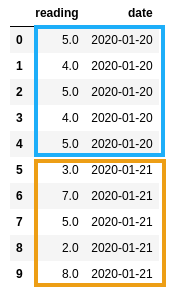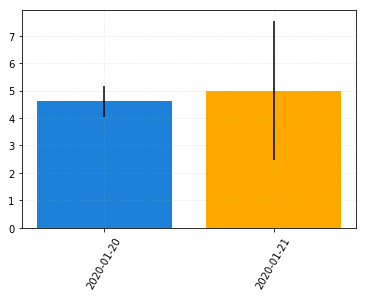Matplotlib Errorbar Examples

# Matplotlib Errorbar Examples

Last updated:

WIP Alert This is a work in progress. Current information is correct but more content may be added in the future.

All code can be found on this jupyter notebook

## Bar plot with error bars

The correct name is standard error because we're calculating the standard deviation of a sample.

Extract the mean and std deviation (as a proxy for error) from the data and use plt.bar() to plot the bars and then plt.vlines() to plot the vertical lines:

import pandas as pd
import numpy as np
import matplotlib.pyplot as plt

df = pd.DataFrame({
"reading": [5.0, 4.0, 5.0, 4.0, 5.0, 3.0, 7.0, 5.0, 2.0, 8.0],
"date": [
"2020-01-20",
"2020-01-20",
"2020-01-20",
"2020-01-20",
"2020-01-20",
"2020-01-21",
"2020-01-21",
"2020-01-21",
"2020-01-21",
"2020-01-21"
]})

# aggregate data by date

xs = range(len(df_grouped['mean'].values))
ys = df_grouped['mean'].values

labels = df_grouped['date'].values

plt.bar(xs, ys)
plt.vlines(xs,
df_grouped['mean'] - df_grouped['std'],
df_grouped['mean'] + df_grouped['std'])5 readings for Jan 20, andNote that the bat plots indicate similar
TODO